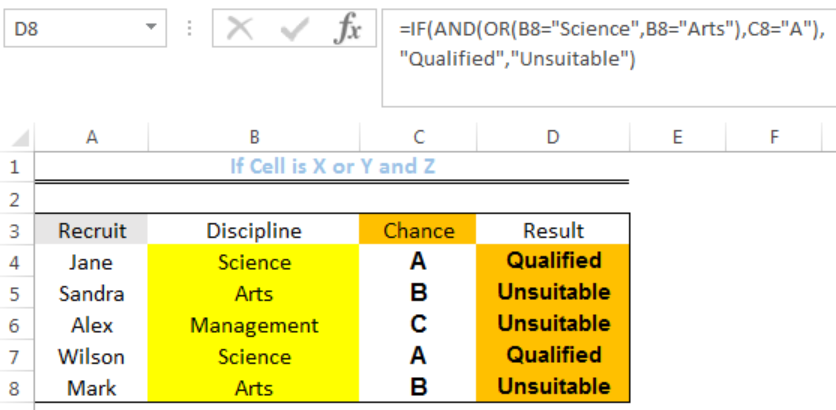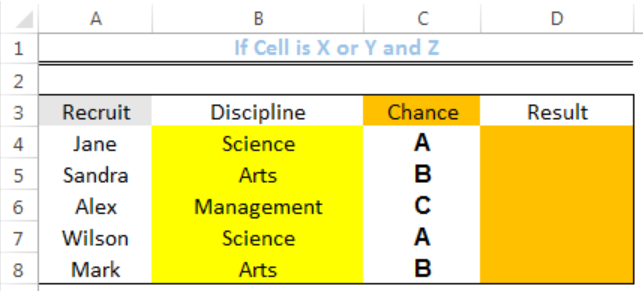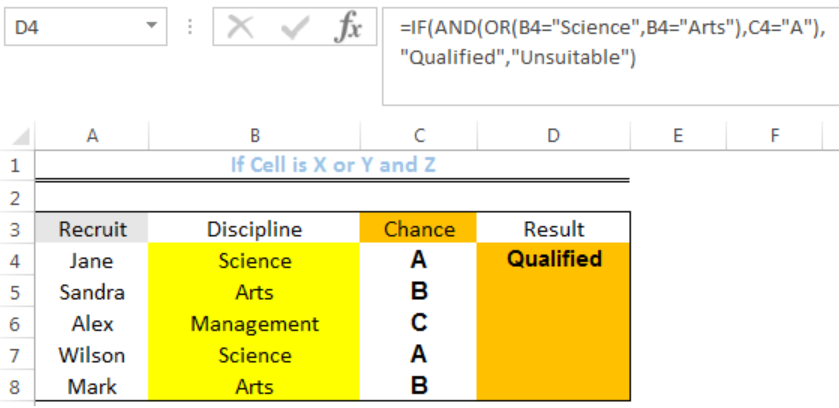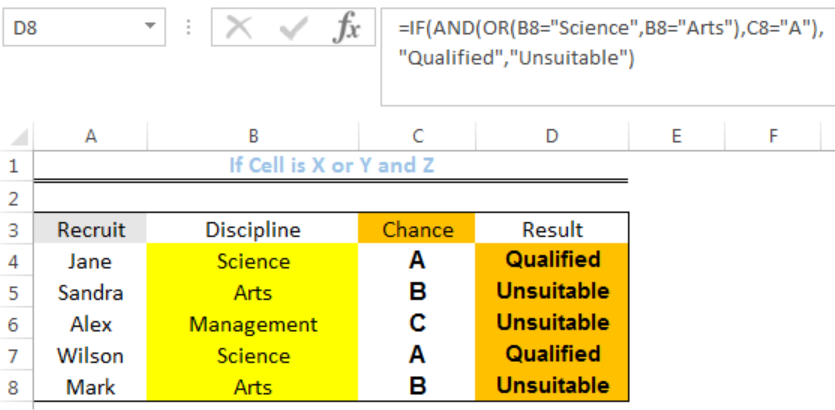Get instant live expert help with Excel or Google Sheets“My Excelchat expert helped me in less than 20 minutes, saving me what would have been 5 hours of work!”

#### Post your problem and you’ll get Expert help in seconds.

Your message must be at least 40 characters
Our professional Expert are available now. Your privacy is guaranteed.

# If Cell is X or Y and Z

We can use a nested formula that combines the IF, AND, and OR functions to test for multiple conditions. Here, we will test if a cell value fulfills X OR Y conditions and Z CONDITION. The steps below will walk through the process.Figure 1: Result of If Cell is “Science” or “Arts” and “A”

## General Formula

`= IF(AND(OR(x is True, y is True), z is True),value_if_True,value_if_False)`

The TRUE Value is returned only if the X OR Y CRITERIA IS TRUE and Z IS ALSO TRUE

## Formula

`=IF(AND(OR(B4="Science",B4="Arts"),C4="A"),"Qualified","Unsuitable")`

## Setting up the Data

• We will set up the data by inputting the Names of Recruits in Column A
• The discipline of the recruits are placed in Column B
• Their chances of been hired are placed in Column C with assigned alphabets
• Column D is where we want the formula to return the result. Qualified signifies a TRUE value and Unsuitable is a FALSE valueFigure 2: Setting up the Data

## If Cell is “Science” or “Arts” and “A”

• We will click on Cell D4
• We will insert the formula below into the cell
`=IF(AND(OR(B4="Science",B4="Arts"),C4="A"),"Qualified","Unsuitable")`
• We will press the enter keyFigure 3: If Cell is “Science” or “Arts” and “A”

• We will click on Cell D4 again
• We will double-click on the fill handle (the small plus sign at the bottom right of Cell D4) and drag down to copy the formula into the other cellsFigure 4: Result of If Cell is “Science” or “Arts” and “A”

## Explanation

`=IF(AND(OR(B4="Science",B4="Arts"),C4="A"),"Qualified","Unsuitable")`

The OR function checks Cell B4 if it has either science or arts as its value. The AND function checks if Cell C4 has the value, “A.” The IF function returns the result as Qualified or Unsuitable if the criteria are met.

## Note

• If we chose to only leave the inverted commas and remove “Unsuitable” from the formula, we will have empty cells in the place of Unsuitable as the result.
• If we remove the False value (UNSUITABLE) including the inverted commas, we will have FALSE in the place of Unsuitable as the result.

## Instant Connection to an Expert through our Excelchat Service

Most of the time, the problem you will need to solve will be more complex than a simple application of a formula or function. If you want to save hours of research and frustration, try our live Excelchat service! Our Excel Experts are available 24/7 to answer any Excel question you may have. We guarantee a connection within 30 seconds and a customized solution within 20 minutes.

Solution examplesI have a list or people who are ranked in numbers from 3 to 6 I need to recognize the contents of each multiple cells and create an equivalent letter. for that value into another column. e.g. Cell E1 = 3 to show in new cell that row (H1) the letter "C" I have multiple rows with different values in column "E" Can this be done?
Solved by I. J. in 30 minsHello good afternoon, I have a problem with excel. I need a formula to compare data between 3 columns and give me the result in a fourth column
Solved by X. B. in 58 minsI want to display a range of cells if they meet a specific criteria. I want to display a name IF it does not equal one of three options AND there are any numbers contained in a range of cells
Solved by G. F. in 60 minscan you teach me the steps to create a nested if function, and to nest an AND function inside of an IF function?
Solved by A. Q. in 22 minsCould you help me by explaining the IF function?
Solved by G. L. in 24 mins